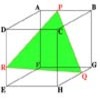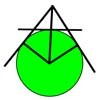#### You may also like### Cut Cube

Find the shape and symmetries of the two pieces of this cut cube.### Mean Geometrically

A and B are two points on a circle centre O. Tangents at A and B cut at C. CO cuts the circle at D. What is the relationship between areas of ADBO, ABO and ACBO?### Logosquares

Ten squares form regular rings either with adjacent or opposite vertices touching. Calculate the inner and outer radii of the rings that surround the squares.

# Rose

##### Age 16 to 18Challenge Level

Why do this problem?
Groups play a key role in understanding the underlying patterns in geometric art as well as underpinning arithmetic, algebra, geometry and being useful in many applications of mathematics.

This challenge is structured to enable learners to find out for themselves about the group of symmetries of a regular pentagon and to find all of its subgroups.They don't have to have any prior knowledge of groups and they do not need to know the axioms of a group because with transformations there is always an inverse. They only need to know that they are looking for sets of transformations which are closed under the operation of taking combinations.

Possible approach
The class could start by listing the symmetries in the four diagrams and discussing how to use symbols for the transformations. Class discussion can then explore the key ideas:
(1) a combination of two reflections in intersecting lines gives a rotation and so once a group contains two reflections it must contain all the symmetries of the pentagon
(2) a group that contains a reflection and a rotation contains all the symmetries of the pentagon
You can introduce the words 'subgroup' and 'isomorphic groups' as the standard terminology for what the learners have discovered for themselves.

Key Questions
What happens when you combine two reflections in intersecting mirror lines?
What transformation results when you combine a reflection in a mirror line and a rotation about a point on the mirror line?
What is the same and what is different about the groups with two elements that contain the identity and reflection in a single mirror line?

Possible extension
The challenge is so structured that it could be a short activity leading on to a similar investigation of the symmetries of a cylinder and finding out why there are only seven frieze groups. See Paint Rollers for Frieze Patterns.

To explore the ideas of groups of transformations further you could read the article Grouping Transformations.

Possible support
The three challenges: Decoding Transformations, Combining Transformations and Simplifying Transformations introduce the idea of an 'algebra' to record transformations and the way transformations combine to give other transformations.